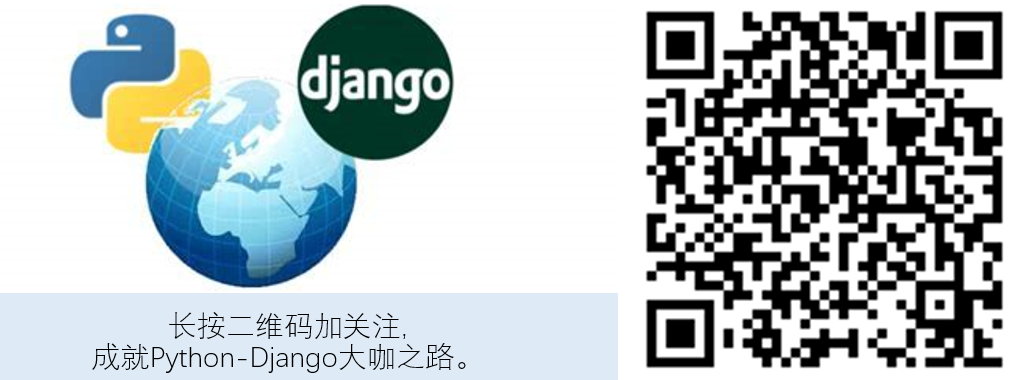# Django模型详解

## 目录

Model (模型) 简而言之即数据模型，是一个Django应用的核心。模型不是数据本身（比如数据表里的数据), 而是抽象的描述数据的构成和逻辑关系。

## 模型定义小案例

``````# models.py
from django.db import models

class Publisher(models.Model):
name = models.CharField(max_length=30)

def __str__(self):
return self.name

class Book(models.Model):
name = models.CharField(max_length=30)
description = models.TextField(blank=True, null=True)
publisher = ForeignKey(Publisher)

def __str__(self):
return self.name
``````

• `CharField`里的`max_length`选项没有定义

• `ForeignKey(Publisher)`里的`on_delete`选项有没有定义

• 这个Field是否有必选项, 比如`CharField``max_length``ForeignKey``on_delete`选项是必须要设置的。

• 这个Field是否必需(blank = True or False)，是否可以为空 (null = True or False)。这关系到数据的完整性。

``````# models.py
from django.db import models

class Publisher(models.Model):
name = models.CharField(max_length=30)

def __str__(self):
return self.name

class Book(models.Model):
name = models.CharField(max_length=30)
description = models.TextField(blank=True, default='')

def __str__(self):
return self.name
``````

## 模型的组成

• 定义的模型字段：包括基础字段和关系字段
• 自定义的Manager方法：改变模型
• `class Meta选项`: 包括排序、索引等等(可选)。
• `def __str__()`：定义单个模型实例对象的名字(可选)。
• `def save()`：重写save方法(可选)。
• `def get_absolute_url()`：为单个模型实例对象生成独一无二的url(可选)
• 其它自定义的方法。

## 模型的字段

`models.Model`提供的常用模型字段包括基础字段和关系字段。

### 基础字段

**CharField() **

**TextField() **

**DateField() 和DateTimeField() **

• 对于DateTimeField: default=timezone.now - 先要`from django.utils import timezone`
• 如果希望自动记录一次修改日期(modified)，可以设置: `auto_now=True`
• 如果希望自动记录创建日期(created),可以设置`auto_now_add=True`

**EmailField() **

IntegerField(), SlugField(), URLField()，BooleanField()

• max_length = xxxx：文件最大长度

• upload_to = “/some folder/”: 指定上传图片路径

### 关系字段

OneToOneField(to, on_delete=xxx, options) - 单对单关系

• to必需指向其他模型，比如 Book or ‘self’ .
• 必需指定`on_delete`选项(删除选项): i.e, “`on_delete = models.CASCADE`” or “`on_delete = models.SET_NULL`” .
• 可以设置 “`related_name = xxx`” 便于反向查询。

ForeignKey(to, on_delete=xxx, options) - 单对多关系

• to必需指向其他模型，比如 Book or ‘self’ .
• 必需指定`on_delete`选项(删除选项): i.e, “`on_delete = models.CASCADE`” or “`on_delete = models.SET_NULL`” .
• 可以设置”default = xxx” or “null = True” ;
• 如果有必要，可以设置 “`limit_choices_to =` “,
• 可以设置 “`related_name = xxx`” 便于反向查询。

ManyToManyField(to, options) - 多对多关系

• to 必需指向其他模型，比如 User or ‘self’ .
• 设置 “`symmetrical = False` “ 表示多对多关系不是对称的，比如A关注B不代表B关注A
• 设置 “`through = 'intermediary model'` “ 如果需要建立中间模型来搜集更多信息。
• 可以设置 “`related_name = xxx`” 便于反向查询。

``````from django.db import models

class Person(models.Model):
name = models.CharField(max_length=128)

def __str__(self):
return self.name

class Group(models.Model):
name = models.CharField(max_length=128)
members = models.ManyToManyField(Person, through='Membership')

def __str__(self):
return self.name

class Membership(models.Model):
date_joined = models.DateField()
invite_reason = models.CharField(max_length=64)
``````

### on_delete删除选项

Django提供了如下几种关联外键删除选项, 可以根据实际需求使用。

• `CASCADE`：级联删除。当你删除publisher记录时，与之关联的所有 book 都会被删除。
• `PROTECT`: 保护模式。如果有外键关联，就不允许删除，删除的时候会抛出ProtectedError错误，除非先把关联了外键的记录删除掉。例如想要删除publisher，那你要把所有关联了该publisher的book全部删除才可能删publisher。
• `SET_NULL`: 置空模式。删除的时候，外键字段会被设置为空。删除publisher后，book 记录里面的publisher_id 就置为null了。
• `SET_DEFAULT`: 置默认值，删除的时候，外键字段设置为默认值。
• `SET()`: 自定义一个值。
• `DO_NOTHING`：什么也不做。删除不报任何错，外键值依然保留，但是无法用这个外键去做查询。

`related_name`用于设置模型反向查询的名字，非常有用。在文初的`Publisher``Book`模型里，我们可以通过`book.publisher`获取每本书的出版商信息，这是因为`Book`模型里有`publisher`这个字段。但是`Publisher`模型里并没有`book`这个字段，那么我们如何通过出版商反查其出版的所有书籍信息呢？

Django对于关联字段默认使用`模型名_set`进行反查，即通过`publisher.book_set.all`查询。但是`book_set`并不是一个很友好的名字，我们更希望通过`publisher.books`获取一个出版社已出版的所有书籍信息，这时我们就要修改我们的模型了，将`related_name`设为`books`, 如下所示：

``````# models.py
from django.db import models

class Publisher(models.Model):
name = models.CharField(max_length=30)

def __str__(self):
return self.name

# 将related_name设置为books
class Book(models.Model):
name = models.CharField(max_length=30)
description = models.TextField(blank=True, default='')

def __str__(self):
return self.name
``````

1. 设置`related_name`前：`publisher.book_set.all`
2. 设置`related_name`后：`publisher.books.all`

## 模型的META选项

• `abstract=True`: 指定该模型为抽象模型
• `proxy=True`: 指定该模型为代理模型
• `verbose_name=xxx``verbose_name_plural=xxx`: 为模型设置便于人类阅读的别名
• `db_table= xxx`: 自定义数据表名
• `odering=['-pub-date']`: 自定义按哪个字段排序，`-`代表逆序
• `permissions=[]`: 为模型自定义权限
• `managed=False`: 默认为True，如果为False，Django不会为这个模型生成数据表
• `indexes=[]`: 为数据表设置索引，对于频繁查询的字段，建议设置索引
• `constraints=`: 给数据库中的数据表增加约束。

## 模型的方法

### 标准方法

• `def __str__()`：给单个模型对象实例设置人为可读的名字(可选)。
• `def save()`：重写save方法(可选)。
• `def get_absolute_url()`：为单个模型实例对象生成独一无二的url(可选)

### 示例一：自定义方法

``````# 为每篇文章生成独一无二的url
def get_absolute_url(self):
return reverse('blog:article_detail', args=[str(self.id)])

# 计数器
def viewed(self):
self.views += 1
self.save(update_fields=['views'])
``````

### 示例二：自定义Manager方法

``````# First, define the Manager subclass.
class DahlBookManager(models.Manager):
def get_queryset(self):
return super().get_queryset().filter(author='Roald Dahl')

# Then hook it into the Book model explicitly.
class Book(models.Model):
title = models.CharField(max_length=100)
author = models.CharField(max_length=50)

objects = models.Manager() # The default manager.
dahl_objects = DahlBookManager() # The Dahl-specific manager.
``````

## 完美的高级Django模型示例

``````from django.db import models
from django.urls import reverse

# 自定义Manager方法
class HighRatingManager(models.Manager):
def get_queryset(self):
return super().get_queryset().filter(rating=1)

# CHOICES选项
class Rating(models.IntegerChoices):
VERYGOOD = 1, 'Very Good'
GOOD = 2, 'Good'

class Product(models.Model):
# 数据表字段
name = models.CharField('name', max_length=30)
rating = models.IntegerField(max_length=1, choices=Rating.choices)

# MANAGERS方法
objects = models.Manager()
high_rating_products =HighRatingManager()

# META类选项
class Meta:
verbose_name = 'product'
verbose_name_plural = 'products'

# __str__方法
def __str__(self):
return self.name

# 重写save方法
def save(self, *args, **kwargs):
do_something()
super().save(*args, **kwargs)
do_something_else()

# 定义单个对象绝对路径
def get_absolute_url(self):
return reverse('product_details', kwargs={'pk': self.id})

# 其它自定义方法
def do_something(self):
``````

## 小结